# 2. Transportation Problem.pptx

29. May 2023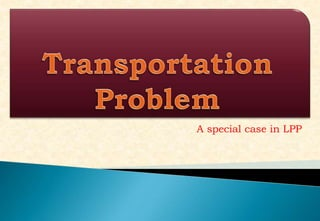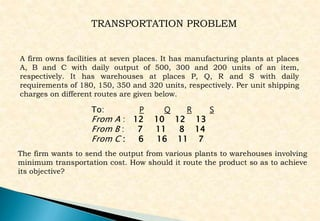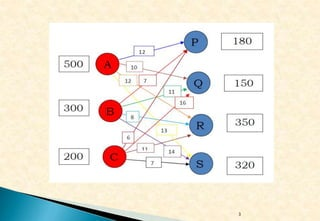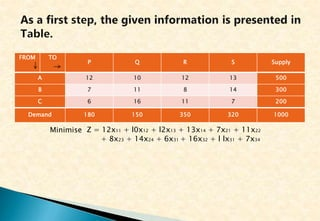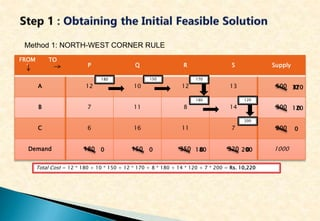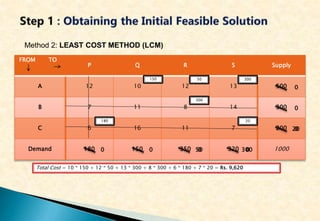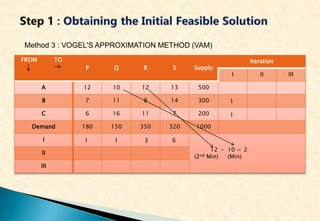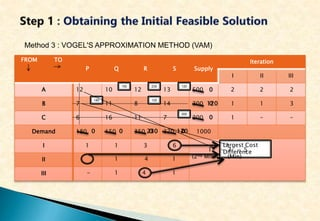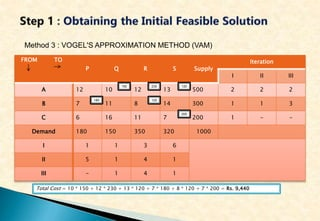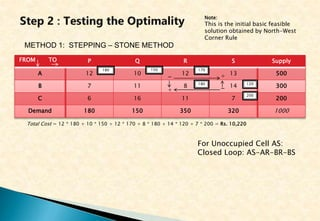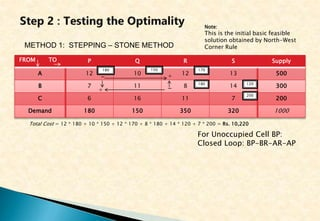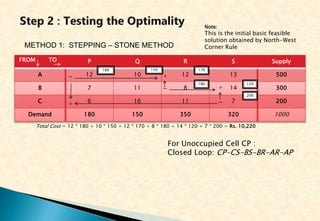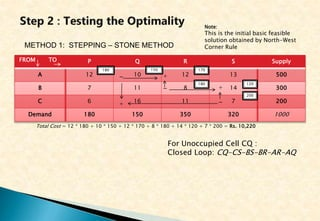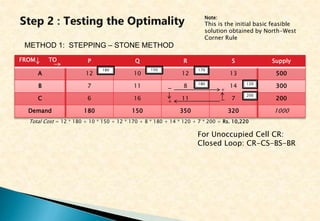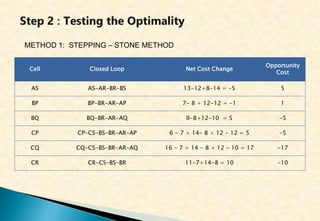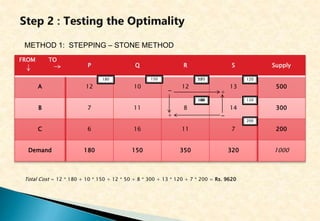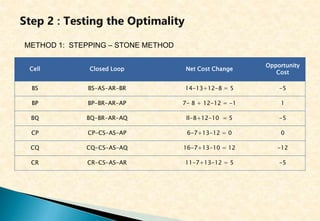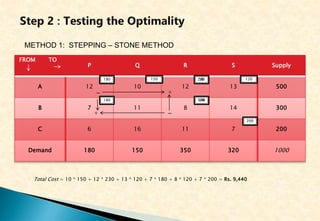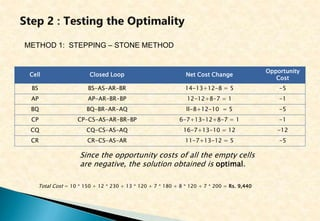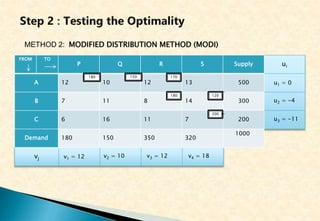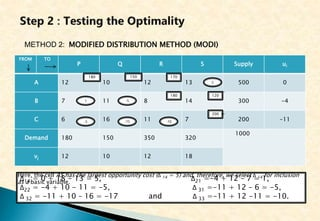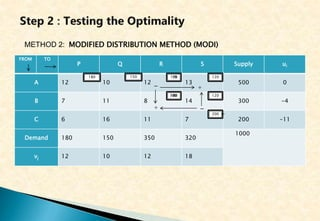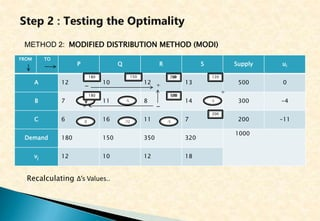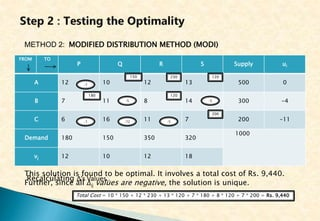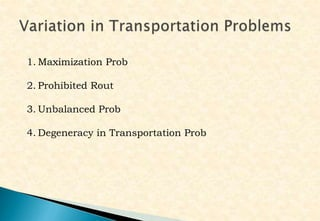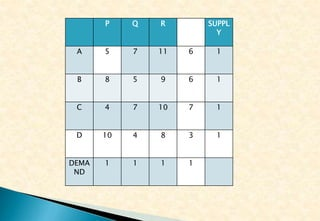1 von 27

### 2. Transportation Problem.pptx

• 1. A special case in LPP
• 2. A firm owns facilities at seven places. It has manufacturing plants at places A, B and C with daily output of 500, 300 and 200 units of an item, respectively. It has warehouses at places P, Q, R and S with daily requirements of 180, 150, 350 and 320 units, respectively. Per unit shipping charges on different routes are given below. TRANSPORTATION PROBLEM To: P Q R S From A : 12 10 12 13 From B : 7 11 8 14 From C : 6 16 11 7 The firm wants to send the output from various plants to warehouses involving minimum transportation cost. How should it route the product so as to achieve its objective?
• 3. 3
• 4. FROM TO P Q R S Supply A 12 10 12 13 500 B 7 11 8 14 300 C 6 16 11 7 200 Demand 180 150 350 320 1000 Minimise Z = 12x11 + l0x12 + l2x13 + 13x14 + 7x21 + 11x22 + 8x23 + 14x24 + 6x31 + 16x32 + l lx31 + 7x34
• 5. FROM ` TO P Q R S Supply A 12 10 12 13 500 B 7 11 8 14 300 C 6 16 11 7 200 Demand 180 150 350 320 1000 Method 1: NORTH-WEST CORNER RULE 180 150 200 120 180 170 Total Cost = 12 * 180 + 10 * 150 + 12 * 170 + 8 * 180 + 14 * 120 + 7 * 200 = Rs. 10,220 0 0 180 0 200 0 320 170 0 120 0 0
• 6. FROM TO P Q R S Supply A 12 10 12 13 500 B 7 11 8 14 300 C 6 16 11 7 200 Demand 180 150 350 320 1000 Method 2: LEAST COST METHOD (LCM) 180 150 20 300 300 50 Total Cost = 10 * 150 + 12 * 50 + 13 * 300 + 8 * 300 + 6 * 180 + 7 * 20 = Rs. 9,620 0 300 20 0 50 0 0 0 0 0
• 7. FROM TO P Q R S Supply Iteration I II III A 12 10 12 13 500 B 7 11 8 14 300 C 6 16 11 7 200 Demand 180 150 350 320 1000 I II III Method 3 : VOGEL'S APPROXIMATION METHOD (VAM) 12 (2nd Min) - 10 (Min) = 2 1 1 6 3 1 1
• 8. FROM TO P Q R S Supply Iteration I II III A 12 10 12 13 500 2 B 7 11 8 14 300 1 C 6 16 11 7 200 1 Demand 180 150 350 320 1000 I 1 1 3 6 II III Method 3 : VOGEL'S APPROXIMATION METHOD (VAM) 180 120 230 150 200 Largest Cost Difference 0 120 0 120 230 0 0 12 (2nd Min) - 7 (Min) = 5 120 0 0 0 1 4 1 - 1 2 3 - 2 - 1 1 4
• 9. FROM TO P Q R S Supply Iteration I II III A 12 10 12 13 500 2 2 2 B 7 11 8 14 300 1 1 3 C 6 16 11 7 200 1 - - Demand 180 150 350 320 1000 I 1 1 3 6 II 5 1 4 1 III - 1 4 1 Method 3 : VOGEL'S APPROXIMATION METHOD (VAM) 180 120 230 150 200 120 Total Cost = 10 * 150 + 12 * 230 + 13 * 120 + 7 * 180 + 8 * 120 + 7 * 200 = Rs. 9,440
• 10. FROM TO P Q R S Supply A 12 10 12 13 500 B 7 11 8 14 300 C 6 16 11 7 200 Demand 180 150 350 320 1000 METHOD 1: STEPPING – STONE METHOD 180 150 200 120 180 170 Total Cost = 12 * 180 + 10 * 150 + 12 * 170 + 8 * 180 + 14 * 120 + 7 * 200 = Rs. 10,220 Note: This is the initial basic feasible solution obtained by North-West Corner Rule - + - + For Unoccupied Cell AS: Closed Loop: AS-AR-BR-BS
• 11. FROM TO P Q R S Supply A 12 10 12 13 500 B 7 11 8 14 300 C 6 16 11 7 200 Demand 180 150 350 320 1000 METHOD 1: STEPPING – STONE METHOD 180 150 200 120 180 170 Total Cost = 12 * 180 + 10 * 150 + 12 * 170 + 8 * 180 + 14 * 120 + 7 * 200 = Rs. 10,220 Note: This is the initial basic feasible solution obtained by North-West Corner Rule + - For Unoccupied Cell BP: Closed Loop: BP-BR-AR-AP - +
• 12. FROM TO P Q R S Supply A 12 10 12 13 500 B 7 11 8 14 300 C 6 16 11 7 200 Demand 180 150 350 320 1000 METHOD 1: STEPPING – STONE METHOD 180 150 200 120 180 170 Total Cost = 12 * 180 + 10 * 150 + 12 * 170 + 8 * 180 + 14 * 120 + 7 * 200 = Rs. 10,220 Note: This is the initial basic feasible solution obtained by North-West Corner Rule + - For Unoccupied Cell CP : Closed Loop: CP-CS-BS-BR-AR-AP - + + -
• 13. FROM TO P Q R S Supply A 12 10 12 13 500 B 7 11 8 14 300 C 6 16 11 7 200 Demand 180 150 350 320 1000 METHOD 1: STEPPING – STONE METHOD 180 150 200 120 180 170 Total Cost = 12 * 180 + 10 * 150 + 12 * 170 + 8 * 180 + 14 * 120 + 7 * 200 = Rs. 10,220 Note: This is the initial basic feasible solution obtained by North-West Corner Rule + - For Unoccupied Cell CQ : Closed Loop: CQ-CS-BS-BR-AR-AQ - + + -
• 14. FROM TO P Q R S Supply A 12 10 12 13 500 B 7 11 8 14 300 C 6 16 11 7 200 Demand 180 150 350 320 1000 METHOD 1: STEPPING – STONE METHOD 180 150 200 120 180 170 Total Cost = 12 * 180 + 10 * 150 + 12 * 170 + 8 * 180 + 14 * 120 + 7 * 200 = Rs. 10,220 Note: This is the initial basic feasible solution obtained by North-West Corner Rule - + - + For Unoccupied Cell CR: Closed Loop: CR-CS-BS-BR
• 15. METHOD 1: STEPPING – STONE METHOD Cell Closed Loop Net Cost Change Opportunity Cost AS AS-AR-BR-BS 13-12+8-14 = -5 5 BP BP-BR-AR-AP 7- 8 + 12-12 = -1 1 BQ BQ-BR-AR-AQ ll-8+12-10 = 5 -5 CP CP-CS-BS-BR-AR-AP 6 - 7 + 14- 8 + 12 - 12 = 5 -5 CQ CQ-CS-BS-BR-AR-AQ 16 - 7 + 14 - 8 + 12 - 10 = 17 -17 CR CR-CS-BS-BR 11-7+14-8 = 10 -10
• 16. FROM TO P Q R S Supply A 12 10 12 13 500 B 7 11 8 14 300 C 6 16 11 7 200 Demand 180 150 350 320 1000 METHOD 1: STEPPING – STONE METHOD 180 150 200 120 180 170 Total Cost = 12 * 180 + 10 * 150 + 12 * 50 + 8 * 300 + 13 * 120 + 7 * 200 = Rs. 9620 - + - + 50 300 120
• 17. METHOD 1: STEPPING – STONE METHOD Cell Closed Loop Net Cost Change Opportunity Cost BS BS-AS-AR-BR 14-13+12-8 = 5 -5 BP BP-BR-AR-AP 7- 8 + 12-12 = -1 1 BQ BQ-BR-AR-AQ ll-8+12-10 = 5 -5 CP CP-CS-AS-AP 6-7+13-12 = 0 0 CQ CQ-CS-AS-AQ 16-7+13-10 = 12 -12 CR CR-CS-AS-AR 11-7+13-12 = 5 -5
• 18. FROM TO P Q R S Supply A 12 10 12 13 500 B 7 11 8 14 300 C 6 16 11 7 200 Demand 180 150 350 320 1000 METHOD 1: STEPPING – STONE METHOD 150 200 180 300 50 Total Cost = 10 * 150 + 12 * 230 + 13 * 120 + 7 * 180 + 8 * 120 + 7 * 200 = Rs. 9,440 - + - + 230 120 180 120
• 19. METHOD 1: STEPPING – STONE METHOD Cell Closed Loop Net Cost Change Opportunity Cost BS BS-AS-AR-BR 14-13+12-8 = 5 -5 AP AP-AR-BR-BP 12-12+8-7 = 1 -1 BQ BQ-BR-AR-AQ ll-8+12-10 = 5 -5 CP CP-CS-AS-AR-BR-BP 6-7+13-12+8-7 = 1 -1 CQ CQ-CS-AS-AQ 16-7+13-10 = 12 -12 CR CR-CS-AS-AR 11-7+13-12 = 5 -5 Since the opportunity costs of all the empty cells are negative, the solution obtained is optimal. Total Cost = 10 * 150 + 12 * 230 + 13 * 120 + 7 * 180 + 8 * 120 + 7 * 200 = Rs. 9,440
• 20. ui vj FROM TO P Q R S Supply A 12 10 12 13 500 B 7 11 8 14 300 C 6 16 11 7 200 Demand 180 150 350 320 1000 METHOD 2: MODIFIED DISTRIBUTION METHOD (MODI) 180 150 200 120 180 170 - + u1 = 0 u3 = -11 u2 = -4 v1 = 12 v3 = 12 v2 = 10 v4 = 18
• 21. FROM TO P Q R S Supply ui A 12 10 12 13 500 0 B 7 11 8 14 300 -4 C 6 16 11 7 200 -11 Demand 180 150 350 320 1000 vj 12 10 12 18 METHOD 2: MODIFIED DISTRIBUTION METHOD (MODI) 180 150 200 120 180 170 - + ∆14 = 0 + 18 - 13 = 5, ∆21 =-4 + 12 – 7 = 1, ∆22 = -4 + 10 - 11 = -5, ∆ 31 =-11 + 12 – 6 = -5, ∆ 32 = -11 + 10 – 16 = -17 and ∆ 33 =-11 + 12 -11 = -10. 5 -10 -15 -5 -5 1 Here, the cell AS has the largest opportunity cost (∆ 14 = 5) and, therefore, we select ∆ 14 for inclusion as a basic variable.
• 22. FROM TO P Q R S Supply ui A 12 10 12 13 500 0 B 7 11 8 14 300 -4 C 6 16 11 7 200 -11 Demand 180 150 350 320 1000 vj 12 10 12 18 METHOD 2: MODIFIED DISTRIBUTION METHOD (MODI) 180 150 200 120 180 170 - - + - + 300 50 120
• 23. FROM TO P Q R S Supply ui A 12 10 12 13 500 0 B 7 11 8 14 300 -4 C 6 16 11 7 200 -11 Demand 180 150 350 320 1000 vj 12 10 12 18 METHOD 2: MODIFIED DISTRIBUTION METHOD (MODI) 180 150 200 120 300 50 + -5 -5 -12 0 -5 1 Recalculating ∆’s Values.. - + - + 120 230 180
• 24. FROM TO P Q R S Supply ui A 12 10 12 13 500 0 B 7 11 8 14 300 -4 C 6 16 11 7 200 -11 Demand 180 150 350 320 1000 vj 12 10 12 18 METHOD 2: MODIFIED DISTRIBUTION METHOD (MODI) 180 150 200 120 120 230 -5 -5 -12 -1 -5 -1 Recalculating ∆’s Values.. This solution is found to be optimal. It involves a total cost of Rs. 9,440. Further, since all ∆ij values are negative, the solution is unique. Total Cost = 10 * 150 + 12 * 230 + 13 * 120 + 7 * 180 + 8 * 120 + 7 * 200 = Rs. 9,440
• 25. 1. Maximization Prob 2. Prohibited Rout 3. Unbalanced Prob 4. Degeneracy in Transportation Prob
• 26. P Q R SSS SUPPL Y A 5 7 11 6 1 B 8 5 9 6 1 C 4 7 10 7 1 D 10 4 8 3 1 DEMA ND 1 1 1 1